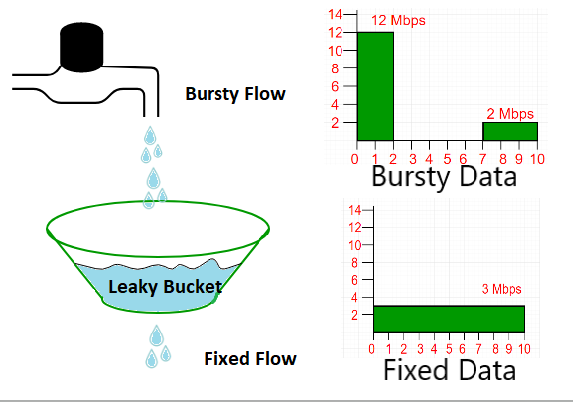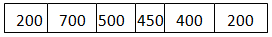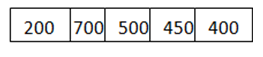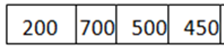Open In App
Related Articles
• Write an Interview Experience
• Computer Network Tutorial

# Computer Network | Leaky bucket algorithm

In the network layer, before the network can make Quality of service guarantees, it must know what traffic is being guaranteed. One of the main causes of congestion is that traffic is often bursty.

To understand this concept first we have to know little about traffic shaping. Traffic Shaping is a mechanism to control the amount and the rate of traffic sent to the network. Approach of congestion management is called Traffic shaping. Traffic shaping helps to regulate the rate of data transmission and reduces congestion.

There are 2 types of traffic shaping algorithms:

1. Leaky Bucket
2. Token Bucket

Suppose we have a bucket in which we are pouring water, at random points in time, but we have to get water at a fixed rate, to achieve this we will make a hole at the bottom of the bucket. This will ensure that the water coming out is at some fixed rate, and also if the bucket gets full, then we will stop pouring water into it.

The input rate can vary, but the output rate remains constant. Similarly, in networking, a technique called leaky bucket can smooth out bursty traffic. Bursty chunks are stored in the bucket and sent out at an average rate.In the above figure, we assume that the network has committed a bandwidth of 3 Mbps for a host. The use of the leaky bucket shapes the input traffic to make it conform to this commitment. In the above figure, the host sends a burst of data at a rate of 12 Mbps for 2s, for a total of 24 Mbits of data. The host is silent for 5 s and then sends data at a rate of 2 Mbps for 3 s, for a total of 6 Mbits of data. In all, the host has sent 30 Mbits of data in 10 s. The leaky bucket smooths out the traffic by sending out data at a rate of 3 Mbps during the same 10 s.

Without the leaky bucket, the beginning burst may have hurt the network by consuming more bandwidth than is set aside for this host. We can also see that the leaky bucket may prevent congestion.

A simple leaky bucket algorithm can be implemented using FIFO queue. A FIFO queue holds the packets. If the traffic consists of fixed-size packets (e.g., cells in ATM networks), the process removes a fixed number of packets from the queue at each tick of the clock. If the traffic consists of variable-length packets, the fixed output rate must be based on the number of bytes or bits.

The following is an algorithm for variable-length packets:

1. Initialize a counter to n at the tick of the clock.
2. Repeat until n is smaller than the packet size of the packet at the head of the queue.
1. Pop a packet out of the head of the queue, say P.
2. Send the packet P, into the network
3. Decrement the counter by the size of packet P.
3. Reset the counter and go to step 1.

Note: In the below examples, the head of the queue is the rightmost position and the tail of the queue is the leftmost position.

Example: Let n=1000

Packet=Since n > size of the packet at the head of the Queue, i.e. n > 200
Therefore, n = 1000-200 = 800
Packet size of 200 is sent into the network.Now, again n > size of the packet at the head of the Queue, i.e. n > 400
Therefore, n = 800-400 = 400
Packet size of 400 is sent into the network.Since, n < size of the packet at the head of the Queue, i.e.  n < 450
Therefore, the procedure is stopped.

Initialise n = 1000 on another tick of the clock.
This procedure is repeated until all the packets are sent into the network.

Below is the implementation of above explained approach:

## C++

 `// cpp program to implement leakybucket``#include ``using` `namespace` `std;``int` `main()``{``    ``int` `no_of_queries, storage, output_pkt_size;``    ``int` `input_pkt_size, bucket_size, size_left;` `    ``// initial packets in the bucket``    ``storage = 0;` `    ``// total no. of times bucket content is checked``    ``no_of_queries = 4;` `    ``// total no. of packets that can``    ``// be accommodated in the bucket``    ``bucket_size = 10;` `    ``// no. of packets that enters the bucket at a time``    ``input_pkt_size = 4;` `    ``// no. of packets that exits the bucket at a time``    ``output_pkt_size = 1;``    ``for` `(``int` `i = 0; i < no_of_queries; i++) ``// space left``    ``{``        ``size_left = bucket_size - storage;``        ``if` `(input_pkt_size <= size_left) {``            ``// update storage``            ``storage += input_pkt_size;``        ``}``        ``else` `{``            ``printf``(``"Packet loss = %d\n"``, input_pkt_size);``        ``}``        ``printf``(``"Buffer size= %d out of bucket size= %d\n"``,``               ``storage, bucket_size);``        ``storage -= output_pkt_size;``    ``}``    ``return` `0;``}` `// This code is contributed by bunny09262002``// Improved by: rishitchaudhary`

## Java

 `// Java Implementation of Leaky bucket` `import` `java.io.*;``import` `java.util.*;` `class` `Leakybucket {``    ``public` `static` `void` `main(String[] args)``    ``{``        ``int` `no_of_queries, storage, output_pkt_size;``        ``int` `input_pkt_size, bucket_size, size_left;` `        ``// initial packets in the bucket``        ``storage = ``0``;` `        ``// total no. of times bucket content is checked``        ``no_of_queries = ``4``;` `        ``// total no. of packets that can``        ``// be accommodated in the bucket``        ``bucket_size = ``10``;` `        ``// no. of packets that enters the bucket at a time``        ``input_pkt_size = ``4``;` `        ``// no. of packets that exits the bucket at a time``        ``output_pkt_size = ``1``;``        ``for` `(``int` `i = ``0``; i < no_of_queries; i++) {``            ``size_left = bucket_size - storage; ``// space left` `            ``if` `(input_pkt_size <= (size_left)) {``                ``storage += input_pkt_size;``            ``}``            ``else` `{``                ``System.out.println(``"Packet loss = "``                                   ``+ input_pkt_size);``            ``}``            ``System.out.println(``"Buffer size= "` `+ storage``                               ``+ ``" out of bucket size= "``                               ``+ bucket_size);``            ``storage -= output_pkt_size;``        ``}``    ``}``}``// Improved by: rishitchaudhary`

## Python3

 `# initial packets in the bucket``storage ``=` `0` `# total no. of times bucket content is checked``no_of_queries ``=` `4` `# total no. of packets that can``# be accommodated in the bucket``bucket_size ``=` `10` `# no. of packets that enters the bucket at a time``input_pkt_size ``=` `4` `# no. of packets that exits the bucket at a time``output_pkt_size ``=` `1``for` `i ``in` `range``(``0``, no_of_queries):  ``# space left` `    ``size_left ``=` `bucket_size ``-` `storage``    ``if` `input_pkt_size <``=` `size_left:``      ``# update storage``        ``storage ``+``=` `input_pkt_size``    ``else``:``        ``print``(``"Packet loss = "``, input_pkt_size)` `    ``print``(f``"Buffer size= {storage} out of bucket size = {bucket_size}"``)` `    ``# as packets are sent out into the network, the size of the storage decreases``    ``storage ``-``=` `output_pkt_size`  `# This code is contributed by Arpit Jain``# Improved by: rishitchaudhary`

## C#

 `// C# Implementation of Leaking bucket``using` `System;` `class` `Leakingbucket``{``  ``static` `void` `Main(``string``[] args)``  ``{``    ``int` `no_of_queries, storage, output_pkt_size;``    ``int` `input_pkt_size, bucket_size, size_left;` `    ``// initial packets in the bucket``    ``storage = 0;` `    ``// total no. of times bucket content is checked``    ``no_of_queries = 4;` `    ``// total no. of packets that can``    ``// be accommodated in the bucket``    ``bucket_size = 10;` `    ``// no. of packets that enters the bucket at a time``    ``input_pkt_size = 4;` `    ``// no. of packets that exits the bucket at a time``    ``output_pkt_size = 1;``    ``for` `(``int` `i = 0; i < no_of_queries; i++)``    ``{``      ``size_left = bucket_size - storage; ``// space left` `      ``if` `(input_pkt_size <= (size_left))``      ``{``        ``storage += input_pkt_size;``      ``}``      ``else``      ``{``        ``Console.WriteLine(``"Packet loss = "` `+ input_pkt_size);``      ``}``      ``Console.WriteLine(``"Buffer size= "` `+ storage + ``" out of bucket size= "` `+ bucket_size);``      ``storage -= output_pkt_size;``    ``}``  ``}``}` `// This code is Contributed by phasing17`

## Javascript

 `// JS code to implement the approach` `// initial packets in the bucket``let storage = 0;` `// total no. of times bucket content is checked``let noOfQueries = 4;` `// total no. of packets that can``// be accommodated in the bucket``let bucketSize = 10;` `// no. of packets that enters the bucket at a time``let inputPktSize = 4;` `// no. of packets that exits the bucket at a time``let outputPktSize = 1;` `for` `(let i = 0; i < noOfQueries; i++) { ``// space left` `let sizeLeft = bucketSize - storage;``if` `(inputPktSize <= sizeLeft) {``// update storage``storage += inputPktSize;``} ``else` `{``console.log(``"Packet loss = "``, inputPktSize);``}` `console.log(`Buffer size= \${storage} out of bucket size = \${bucketSize}`);` `// as packets are sent out into the network, the size of the storage decreases``storage -= outputPktSize;``}` `// This code is contributed by phasing17`

Output

```Buffer size= 4 out of bucket size= 10
Buffer size= 7 out of bucket size= 10
Buffer size= 10 out of bucket size= 10
Packet loss = 4
Buffer size= 9 out of bucket size= 10```

Difference between Leaky and Token buckets –

Some advantage of token Bucket over leaky bucket

• If a bucket is full in tokens Bucket, tokens are discard not packets. While in leaky bucket, packets are discarded.
• Token Bucket can send large bursts at a faster rate while leaky bucket always sends packets at constant rate.
• Predictable Traffic Shaping: Token Bucket offers more predictable traffic shaping compared to leaky bucket. With token bucket, the network administrator can set the rate at which tokens are added to the bucket, and the maximum number of tokens that the bucket can hold. This allows for better control over the network traffic and can help prevent congestion.
• Better Quality of Service (QoS): Token Bucket provides better QoS compared to leaky bucket. This is because token bucket can prioritize certain types of traffic by assigning different token arrival rates to different classes of packets. This ensures that important packets are sent first, while less important packets are sent later, helping to ensure that the network runs smoothly.
• More efficient use of network bandwidth: Token Bucket allows for more efficient use of network bandwidth as it allows for larger bursts of data to be sent at once. This can be useful for applications that require high-speed data transfer or for streaming video content.
• More granular control: Token Bucket provides more granular control over network traffic compared to leaky bucket. This is because it allows the network administrator to set the token arrival rate and the maximum token count, which can be adjusted according to the specific needs of the network.
• Easier to implement: Token Bucket is generally considered easier to implement compared to leaky bucket. This is because token bucket only requires the addition and removal of tokens from a bucket, while leaky bucket requires the use of timers and counters to determine when to release packets.

Some Disadvantage of token Bucket over leaky bucket

• Tokens may be wasted: In Token Bucket, tokens are generated at a fixed rate, even if there is no traffic on the network. This means that if no packets are sent, tokens will accumulate in the bucket, which could result in wasted resources. In contrast, with leaky bucket, the network only generates packets when there is traffic, which helps to conserve resources.
• Delay in packet delivery: Token Bucket may introduce delay in packet delivery due to the accumulation of tokens. If the token bucket is empty, packets may need to wait for the arrival of new tokens, which can lead to increased latency and packet loss.
• Lack of flexibility: Token Bucket is less flexible compared to leaky bucket in terms of shaping network traffic. This is because the token generation rate is fixed, and cannot be changed easily to meet the changing needs of the network. In contrast, leaky bucket can be adjusted more easily to adapt to changes in network traffic.
• Complexity: Token Bucket can be more complex to implement compared to leaky bucket, especially when different token generation rates are used for different types of traffic. This can make it more difficult for network administrators to configure and manage the network.
• Inefficient use of bandwidth: In some cases, Token Bucket may lead to inefficient use of bandwidth. This is because Token Bucket allows for large bursts of data to be sent at once, which can cause congestion and lead to packet loss. In contrast, leaky bucket helps to prevent congestion by limiting the amount of data that can be sent at any given time.

This article is contributed by Abhishek Kumar and Himanshu Gupta. If you like GeeksforGeeks and would like to contribute, you can also write an article using write.geeksforgeeks.org or mail your article to review-team@geeksforgeeks.org. See your article appearing on the GeeksforGeeks main page and help other Geeks.